Home > CCA2 > Chapter 9 > Lesson 9.1.1 > Problem9-11

9-11.
1. Solve each equation for 0 ≤ θ ≤ 360. You may need your calculator, but remember that your calculator only gives one answer. Most of these problems have more than one answer. Think about all four quadrants. Homework Help ✎

1. sin θ = 0.5

2. cos θ = −0.5

3. 4 tan θ − 4 = 0

4. 3 sin2 θ = 1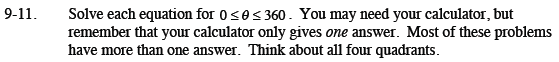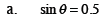Where is the sinθ = 0.5? Remember sin is the y-value. What would θ be at those places?

θ = 30° θ = 150°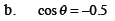Where is the cosθ = −0.5? Remember cos is the x-value. What would θ be at those places?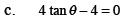Solve for the tanθ. Draw a unit circle. Where is the tanθ of that value?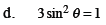Use your calculator to get one of the values. Then use symmetry to get the other 3 values. There are 4 values because when you take the square root of both sides the answer would be plus or minus!